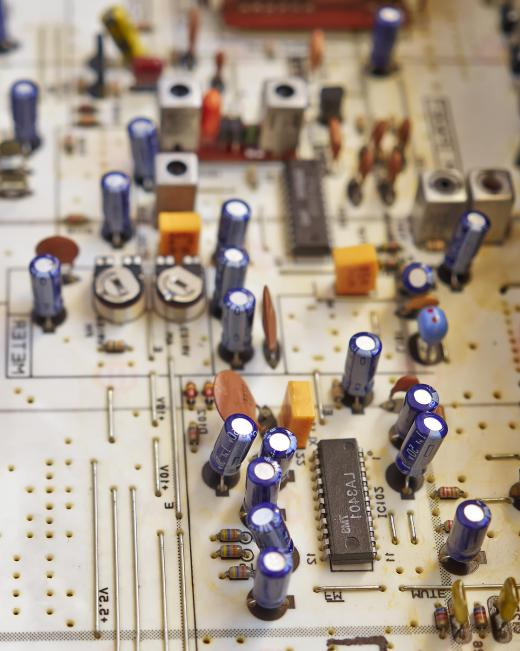Science
Fact Checked

# What Is a RC Circuit?

Geisha A. Legazpi
Geisha A. Legazpi

A resistor-capacitor (RC) circuit is a combination of resistors and capacitors used for filters and timing circuits. In a simple RC circuit, there is a single resistor and a single capacitor. The RC circuit is usually used as an electrical filter that is frequency sensitive. For instance, a low-pass filter will pass the lower frequencies more than other frequencies. In timing circuits, the time for the voltage to rise in the capacitor of an RC circuit is determined by the value of net resistance and capacitance.

Resistors are electrical devices that have a linear relationship between the voltage (V) across them and the current (I) passing through them. Resistance is actually the ratio of the V/I, which means that for the same resistance, a higher voltage across the resistor has a corresponding higher current passing through the resistance. Meanwhile, capacitors are electrical devices that store charges.In timing circuits, capacitance helps determine the time for the voltage to rise in the capacitor of an RC circuit.

In terms of charge (Q) and voltage (V), capacitance is the ratio of Q/V. This means that for the same capacitance, a higher voltage corresponds to higher charge content. It should be noted that current is the rate of charge transfer in coulombs per second, where the coulomb is the unit of electrical charge.

The RC circuit diagram may have a resistor in the input and a capacitor in the output. The circuit current when voltage first appears in the input is dependent on the resistor. When the capacitor charges, the current is near zero. The RC circuit equation defines the RC time constant as the product of resistance and capacitance. A higher time constant means the charge and discharge times will be longer.A resistor is an electronic component that can lower a circuit’s voltage and its flow of electrical current, and a capacitor is a component that can store electrical charges.

A square wave generator may be used to demonstrate the effect of a low time constant. The circuit response is determined by the time constant, and with a high time constant the rise and fall times of the RC circuit output increase. For instance, when the capacitance is zero in a capacitor output RC circuit, the input square wave will also appear in the output as almost the same waveform. When the capacitance in the output is increased, the charging time of the capacitor will make the output look more like a triangular output. The actual output of this low-pass circuit will roughly ramp upward and downward when the square wave falls.

There are several types of RC filter circuits. Filters are frequency-selective circuits that may be low-pass, high-pass, and even band-pass type. The parallel RC circuit is commonly used for low-pass filters that extract audio from higher-frequency components such as radio frequency carriers. RC circuits with the resistor in the output are usually the high-pass filters. As the frequency decreases, approaching zero cycles per second, the output of the high-pass filter decreases or rolls off.

## You might also Like